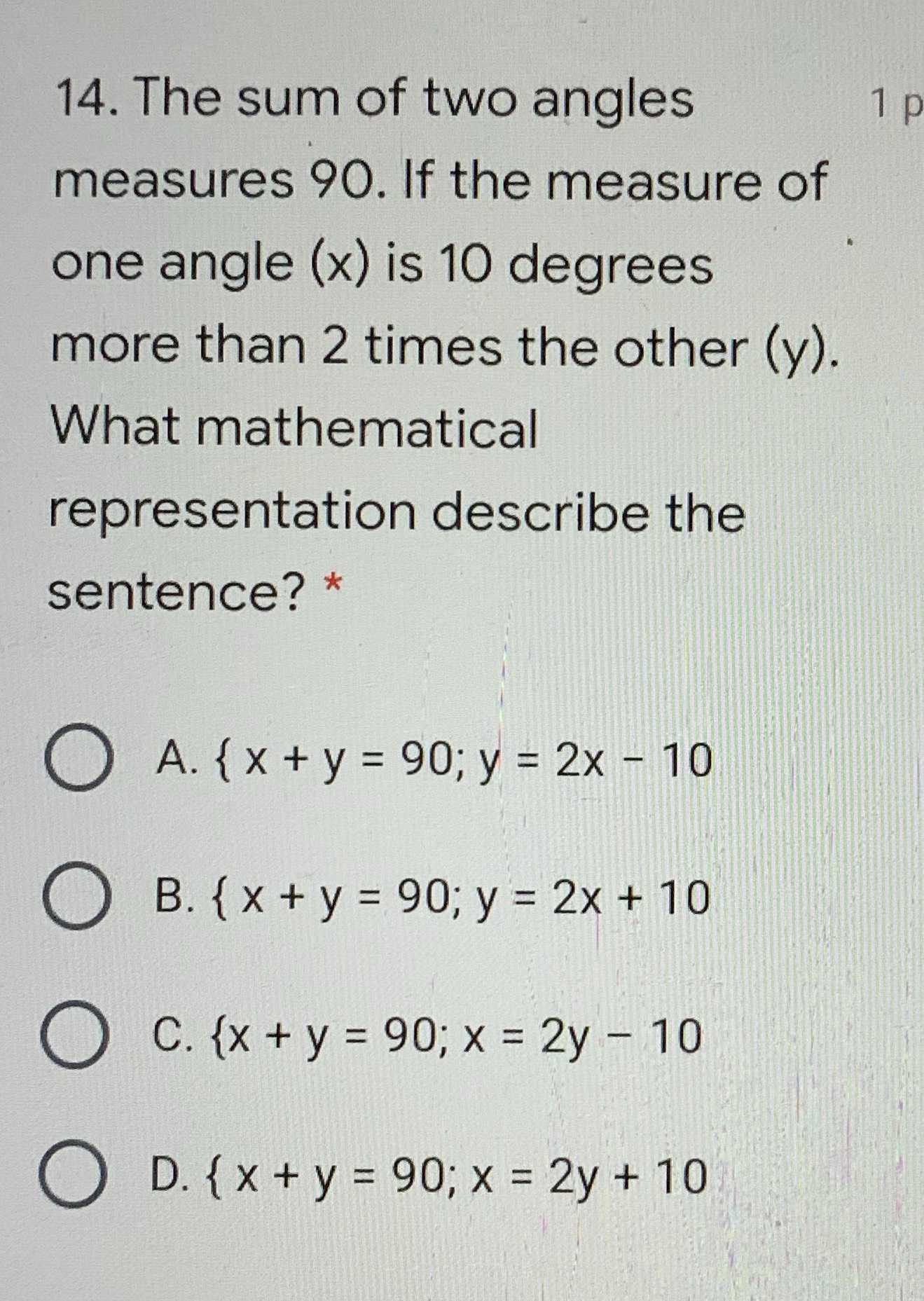### ¿Todavía tienes preguntas de matemáticas?

Pregunte a nuestros tutores expertos
Algebra
Pregunta14. The sum of two angles measures $$90$$ . If the measure of one angle $$( x )$$ is $$10$$ degrees more than $$2$$ times the other $$( y )$$ . What mathematical representation describe the sentence? *

A. $$\{ x + y = 90 ; y = 2 x - 10$$

B. $$\{ x + y = 90 ; y = 2 x + 10$$

C. $$\{ x + y = 90 ; x = 2 y - 10$$

D. $$\{ x + y = 90 ; x = 2 y + 10$$